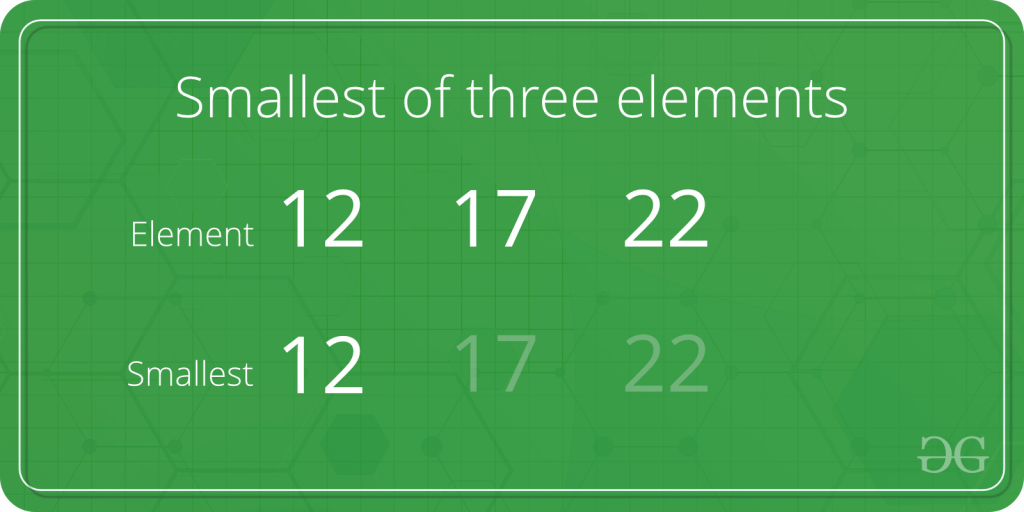# Program to find the smallest element among three elements

Given three numbers. The task is to find the smallest among given three numbers.Examples:

```Input: first = 15, second = 16, third = 10
Output: 10

Input: first = 5, second = 3, third = 6
Output: 3
```

## Recommended: Please try your approach on {IDE} first, before moving on to the solution.

Approach:

1. Check if the first element is smaller than second and third. If yes then print it.
2. Else if check if the second element is smaller than first and third. If yes then print it.
3. Else third is the smallest element and print it.

Below is the implementation of the above approach:

## C++

 `// C++ implementation to find ` `// the smallest of three elements ` `#include ` `using` `namespace` `std; ` ` `  `int` `main() ` `{ ` `    ``int` `a = 5, b = 7, c = 10; ` ` `  `    ``if` `(a <= b && a <= c) ` `        ``cout << a << ``" is the smallest"``; ` ` `  `    ``else` `if` `(b <= a && b <= c) ` `        ``cout << b << ``" is the smallest"``; ` ` `  `    ``else` `        ``cout << c << ``" is the smallest"``; ` ` `  `    ``return` `0; ` `} `

## Java

 `// Java implementation to find ` `// the smallest of three elements ` ` `  `import` `java.io.*; ` ` `  `class` `GFG { ` `   `  ` `  ` `  `    ``public` `static` `void` `main (String[] args) { ` `            ``int` `a = ``5``, b = ``7``, c = ``10``; ` ` `  `    ``if` `(a <= b && a <= c) ` `        ``System.out.println( a + ``" is the smallest"``); ` ` `  `    ``else` `if` `(b <= a && b <= c) ` `        ``System.out.println( b + ``" is the smallest"``); ` ` `  `    ``else` `        ``System.out.println( c + ``" is the smallest"``); ` ` `  `    ``} ` `} ` `// This code is contributed by shs.. `

## Python3

 `# Python implementation to find ` `# the smallest of three elements ` ` `  `a, b, c ``=` `5``, ``7``, ``10` ` `  `if``(a <``=` `b ``and` `a <``=` `c): ` `    ``print``(a, ``"is the smallest"``) ` ` `  `elif``(b <``=` `a ``and` `b <``=` `c): ` `    ``print``(b, ``"is the smallest"``) ` `else``: ` `    ``print``(c, ``"is the smallest"``) ` ` `  `# This code is contributed  ` `# by 29AjayKumar `

## C#

 `// C# implementation to find  ` `// the smallest of three elements ` `using` `System; ` ` `  `class` `GFG ` `{ ` `static` `public` `void` `Main () ` `{ ` `int` `a = 5, b = 7, c = 10;  ` `if` `(a <= b && a <= c)  ` `    ``Console.WriteLine( a + ``" is the smallest"``);  ` ` `  `else` `if` `(b <= a && b <= c)  ` `    ``Console.WriteLine( b + ``" is the smallest"``);  ` ` `  `else` `    ``Console.WriteLine( c + ``" is the smallest"``);  ` `} ` `} ` ` `  `// This code is contributed by jit_t `

## PHP

 `

Output:

```5 is the smallest
```

Time complexity : O(1)

Attention reader! Don’t stop learning now. Get hold of all the important DSA concepts with the DSA Self Paced Course at a student-friendly price and become industry ready.

My Personal Notes arrow_drop_upCheck out this Author's contributed articles.

If you like GeeksforGeeks and would like to contribute, you can also write an article using contribute.geeksforgeeks.org or mail your article to contribute@geeksforgeeks.org. See your article appearing on the GeeksforGeeks main page and help other Geeks.

Please Improve this article if you find anything incorrect by clicking on the "Improve Article" button below.